# An introduction to rotations

We start with a discussion of a rotation for which the axis of rotation is fixed, and this example is used to introduce several of the key concepts. The example also serves to illuminate many of the generalizations that are required for characterizing more elaborate rotations.  To this end, our exposition then turns to proper-orthogonal tensors, and many of their interesting features are shown there. One of the intriguing aspects of rotation tensors is the vast range of representations. These include the Euler angle representation (dating to 1760), quaternionic representations dating to Gauss (1777-1855), Hamilton (1805-1865), and Rodrigues (1795-1851) in a controversial and contested series of discoveries, Cayley’s representation, and many others. The set of representations we discuss is far from exhaustive and we refer the reader to Shuster’s authoritative review  for several others.

Throughout this online resource, we employ a tensorial (dyadic) notation that is widely used in the continuum mechanics community. Its use for describing rotations can be traced to Gibbs (1839-1903) [2, 3]. This notation has many advantages, including transparency in the derivation of angular velocities and clarity in specifying the sets of basis vectors that a given rotation can be considered to transform. We strongly encourage those readers who are unfamiliar with tensors to review the background section on linear algebra where the tensor product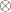of two or more vectors is defined and other tensor operations and properties are discussed.

Contents

## Various representations of a rotation tensor

Dating to Leonhard Euler (1707-1783), we know that rotation tensors and proper-orthogonal tensors are synonymous, and we focus on his representation of a rotation in a form that Gibbs introduced more than a century after Euler’s seminal works. This form is then used to establish related parameterizations including the Euler angle parameterization, the Rodrigues representation, the Cayley representation, and the matrix exponential representation. Specifically, we discuss the following equivalent representations for a rotation tensor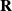associated with a rotation about an axis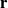through a counterclockwise angle of rotation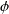:

(1)wheredenotes a fixed basis,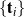is a basis that corotates with a rigid body, the axis of rotation is such thatis a unit vector,

(2)and the Rodrigues vector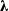, the skew-symmetric Rodrigues tensor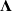, and the skew-symmetric tensor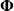are

(3)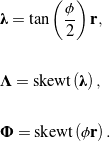It is possible in principle, but often tedious in practice, to transform between a particular representation of a rotation tensor in (1) to another.

## A matrix representation of a rotation

Suppose a rotation tensortransforms a vector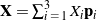into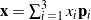, i.e.,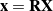. This transformation can be expressed using a matrix representation:

(4)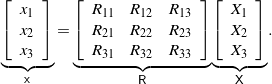The matrix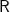has a determinant equal to one and its inverse is its transpose: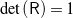and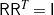, respectively. The identity (4) is known as the active (or alibi) description of a rotation [1, page 494]. In contrast, consider a vector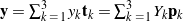. As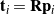, we find that

(5)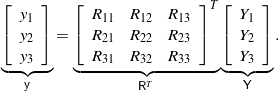This type of identity arises when one set of measurements for a vector is made with respect to the corotational basisthat corresponds to a frame attached to a rigid body and compared to the same vector measured in terms of the fixed basis. The identity (5) is associated with the passive (or alias) description of a rotation [1, page 494]. The presence of the transpose in (5) in contrast to (4) can often be the source of considerable confusion, particularly when expressions from two different sources are being compared.

## Angular velocity vectors

The matrix form is convenient for algebraic manipulations, but one must exercise caution when using matrix representations to compute angular velocities. To this end, our developments of the angular velocity vectors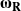and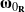associated with a rotation feature the tensor representation of. Several well-known results are examined:

(6)